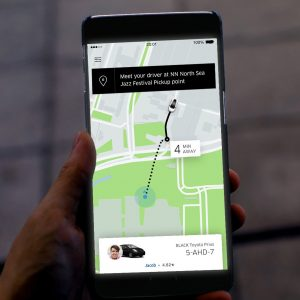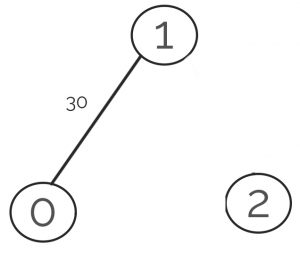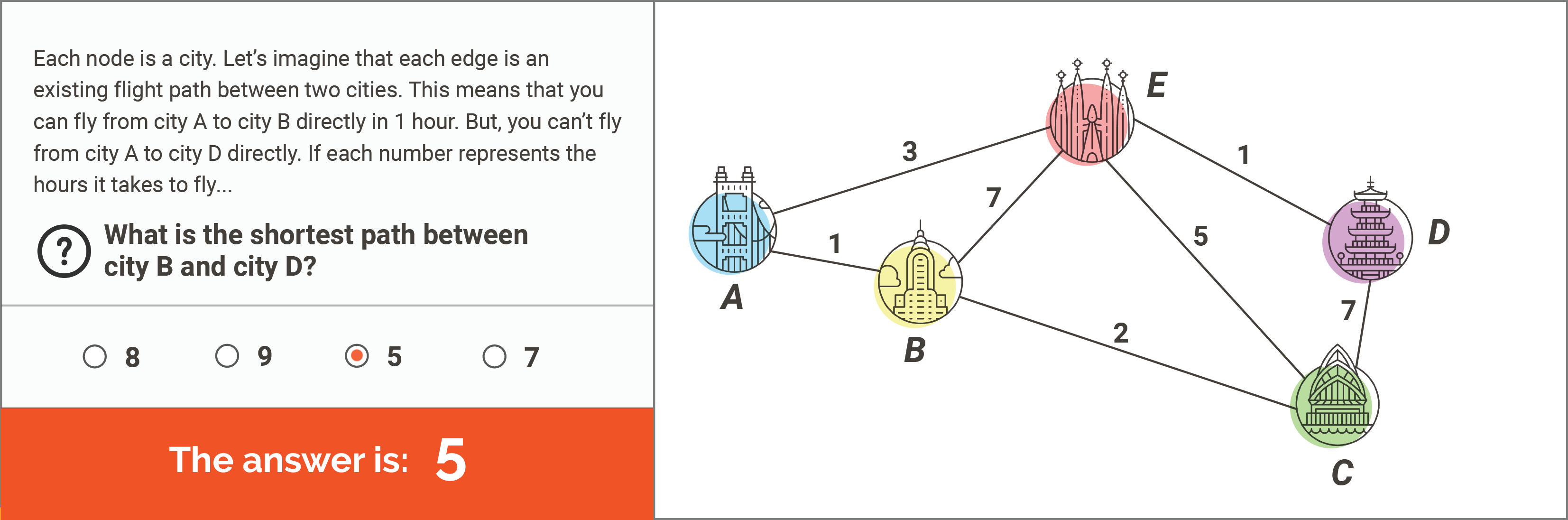# Dijkstra’s Shortest Path Algorithm

“Dijkstra’s Algorithm”. Dijkstra is pronounced “Dike-straw” and Algorithm is pronounced “Al-go-rhythm

When you start understanding what computer science is, it seems both concrete and theoretical. Very mysterious.Apps like Google Maps, or self driving cars, seem very concrete: you can touch them! However, “algorithms”, “data structures”, or “problem solving” can even be done on pen and paper. Well, it turns out, computer science allows you to take abstract ideas and convert them into a way that computers can understand. Let’s take a look at “Dijkstra’s Algorithm” to understand how the world of ideas connects to the world of machines!Dijkstra’s algorithm, like other “Algorithms”, is an idea on how to always efficiently find directions. For example, when you use a ride-sharing app like Uber, it first needs to find the distance between you and nearby cars. However, it’s not going to just draw straight lines on a map between you and each car, because even if a car is close to you it may take a really long time to get to you! Dijkstra’s “Algorithm” takes advantage of the information about the time it takes to go along any road. It is able to use that information to construct the “Shortest Path” between any two intersections. So we call Dijkstra the “Shortest Path Algorithm“. But what really is an algorithm?

##### An algorithm is a set of instructions that can be converted into a computer program.

But what that really means is that an algorithm is an idea that has been converted into mathematical operations.

Now you may say, what? If everything is math, does that mean everything has been converted to numbers? Well yes! We call the information stored for use by algorithm the data, or sometimes the ‘data structure’. So, Dijkstra’s algorithm says that a map needs to be stored as a series of “Nodes” (usually drawn as circles, such as intersections) and “Edges” (usually drawn as lines, such as our roads). So the computer needs to store those as numbers.For example, if you have three nodes, you could number them nodes 0, 1, and 2. And if you had a road that goes from node 0 to node 1 that takes 30 minutes to drive, you might store that as “0 1 30”. Crazy as it may seem, every single thing that a computer does, from social media friends, video game music, to space flight – has to be stored as numbers. And an algorithm is a set of instructions that calculates on those numbers.So what are the actual instructions that allows Dijkstra’s algorithm to find the shortest path? Well let’s pretend we tried to solve this problem, but in English: to find the shortest path between nodes B and D:

###### 1. First, we say that B is “Visited” and all the other nodes are “Unvisited”. 2. Second, let’s say we Assume the distance to A is 1, C is 2, D is unknown, and E is 7. These are just the edge lengths between B and A, B and C, and B and E. We can write that as:[A=1,B=0,C=2,D=?,E=7] . Notice the distance between B and itself is 0. 3. Now let’s pick the first ‘unvisited’ Node with the smallest distance. Well that’s A. 4. Let’s look at all the edges out of A to unvisited nodes, which in this case is just the 3 to E. This means you can get to E through A by adding the edges 1 + 3. And since 1+3=4 is smaller than the current value 7, we change our data structure to this:[A=1,B=0,C=2,D=?,E=4] 5. Now we have finished A and mark it as visited. So what’s the next smallest unvisited node? C=2. 6. C connects to E with 5. So you could go through C to reach E via 2 + 5. However, E is already 4, so we wont change it. 7. C also connects to D with a length of 7, so we can update D to 2 + 7 = 9:[A=1,B=0,C=2,D=9,E=4] 8. Now we mark C as visited, and move on to E 9. E connects to D with length 1, so we can update D to 4+1 = 5:[A=1,B=0,C=2,D=5,E=4] 10. The remaining edges go to A and B which are already visited, so now E is done and can be marked as visited. We move on to D 11. D has no edges to any unvisited nodes, and so this step has us doing nothing. 12. We mark D as visited, and the program terminates because there are no more unvisited nodes. And so we have in our data structure, the values of “Shortest Distance” from B to each node:[A=1,B=0,C=2,D=5,E=4]

Seems pretty long, but what makes this algorithm amazing is how it would work on Any graph of nodes and edges. That means even if you had a million nodes and billion edges, you could still find the shortest path between any two points! For a small graph like the problem above, you might not need to follow such a complicated algorithm. But once you had a big graph, you need a perfect algorithm if you want to guarantee you never make mistakes, and always find the answer within a set amount of time.

However, there is one more important thing about algorithms. Not only does the data need to be stored as numbers, but the instructions need to be converted to machine code as well. That’s why computer programmers don’t write English, they write in a computer programming language such as Java. Here’s Dijkstra’s algorithm written in Java:

With code, the computer is never unsure with what the word “unvisited” or “visited” means, because even those concepts get converted into computations and numbers. In fact, the code itself gets converted to numbers as well when it’s stored inside of the computer in memory. Data is numbers. Code is numbers. With that, computer science allows us to convert real world problems into numbers, and finally allow computers to do those computations really really really fast, all in the blink of an eye.

So when you look around the world and see things, think about how they can be converted into data structures and algorithms, and think about how to solve your problems with algorithms. Before you will know it, you’ll see algorithms everywhere. Some of these were built by people, such as in our everyday devices up to the grandest of industries. Some of these algorithms show up in nature, in physics, in sociology, and economics. The greatest, though, are the undiscovered algorithms, that you or your friends may build to solve problems we don’t know how to solve yet. That’s the beauty of computer science, it allows everyone to precisely describe how to solve problems – regardless of whether the problem originally started in a computer or not!

Dijkstra’s Algorithm is covered in our CS02b – Data Structures class for Advanced students. Click here to try out a class and kick-start your computer science education today!